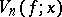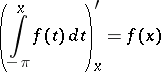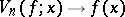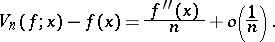# De la Vallée-Poussin singular integral

An integral of the form(see also de la Vallée-Poussin summation method). The sequenceconverges uniformly tofor functionswhich are continuous and-periodic on. Ifat a point, thenas. The following equality is valid :How to Cite This Entry:
De la Vallée-Poussin singular integral. Encyclopedia of Mathematics. URL: http://encyclopediaofmath.org/index.php?title=De_la_Vall%C3%A9e-Poussin_singular_integral&oldid=33425
This article was adapted from an original article by P.P. Korovkin (originator), which appeared in Encyclopedia of Mathematics - ISBN 1402006098. See original article